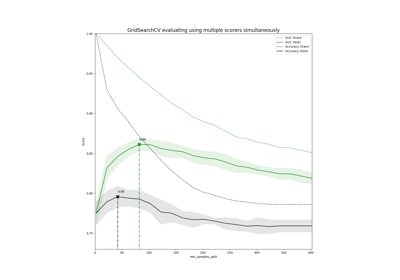# sklearn.metrics.make_scorer¶

sklearn.metrics.make_scorer(score_func, *, greater_is_better=True, needs_proba=False, needs_threshold=False, **kwargs)[source]

Make a scorer from a performance metric or loss function.

This factory function wraps scoring functions for use in GridSearchCV and cross_val_score. It takes a score function, such as accuracy_score, mean_squared_error, adjusted_rand_index or average_precision and returns a callable that scores an estimator’s output.

Read more in the User Guide.

Parameters
score_funccallable,

Score function (or loss function) with signature score_func(y, y_pred, **kwargs).

greater_is_betterboolean, default=True

Whether score_func is a score function (default), meaning high is good, or a loss function, meaning low is good. In the latter case, the scorer object will sign-flip the outcome of the score_func.

needs_probaboolean, default=False

Whether score_func requires predict_proba to get probability estimates out of a classifier.

If True, for binary y_true, the score function is supposed to accept a 1D y_pred (i.e., probability of the positive class, shape (n_samples,)).

needs_thresholdboolean, default=False

Whether score_func takes a continuous decision certainty. This only works for binary classification using estimators that have either a decision_function or predict_proba method.

If True, for binary y_true, the score function is supposed to accept a 1D y_pred (i.e., probability of the positive class or the decision function, shape (n_samples,)).

For example average_precision or the area under the roc curve can not be computed using discrete predictions alone.

Additional parameters to be passed to score_func.

Returns
scorercallable

Callable object that returns a scalar score; greater is better.

Notes

If needs_proba=False and needs_threshold=False, the score function is supposed to accept the output of predict. If needs_proba=True, the score function is supposed to accept the output of predict_proba (For binary y_true, the score function is supposed to accept probability of the positive class). If needs_threshold=True, the score function is supposed to accept the output of decision_function.

Examples

>>> from sklearn.metrics import fbeta_score, make_scorer
>>> ftwo_scorer = make_scorer(fbeta_score, beta=2)
>>> ftwo_scorer
make_scorer(fbeta_score, beta=2)
>>> from sklearn.model_selection import GridSearchCV
>>> from sklearn.svm import LinearSVC
>>> grid = GridSearchCV(LinearSVC(), param_grid={'C': [1, 10]},
...                     scoring=ftwo_scorer)


## Examples using sklearn.metrics.make_scorer¶